Open in App
Not now

# C – Functions

• Difficulty Level : Easy
• Last Updated : 14 Dec, 2022

Functions are sets of statements that take inputs, perform some operations, and produce results. The operation of a function occurs only when it is called.  Rather than writing the same code for different inputs repeatedly, we can call the function instead of writing the same code over and over again. Functions accept parameters, which are data. A function performs a certain action, and it is important for reusing code. Within a function, there are a number of programming statements enclosed by {}.

Example:

`int sum(int a, int b);`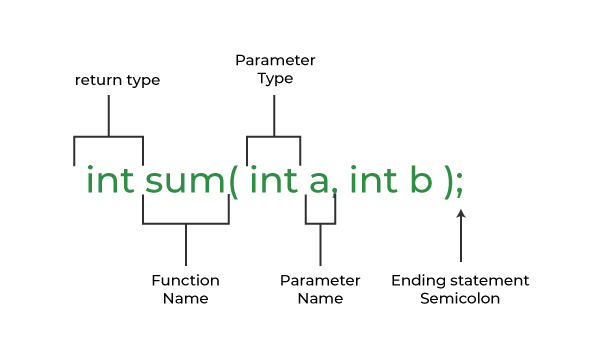## Function Declarations

Function declarations tell the compiler how many parameters a function takes, what kinds of parameters it returns, and what types of data it takes. Function declarations do not need to include parameter names, but definitions must.

Syntax:

`return_type name_of_the_function (parameters); `

Function declaration for the sum of two numbers is shown below

`int sum(int var1, int var2);`

The parameter name is not mandatory while declaring functions. We can also declare the above function without using the name of the data variables.

`int sum(int, int);`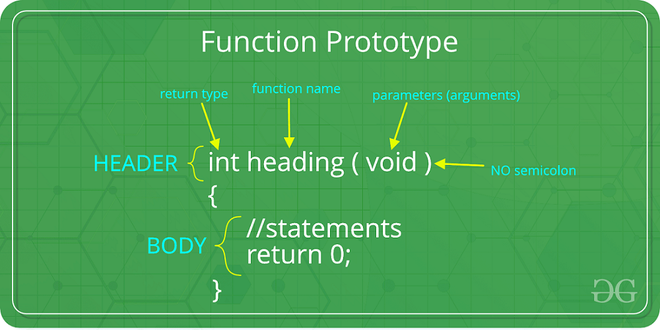## Function Definition

A function definition consists function header and a function body.

```return_type function_name (parameters)
{
//body of the function
}```
• Return_type: The function always starts with a return type of the function. But if there is no return value then the void keyword is used as the return type of the function.
• Function_Name: Name of the function which should be unique.
• Parameters: Values that are passed during the function call.

## Function Call

To Call a function parameters are passed along the function name. In the below example, the first sum function is called and 10,30 are passed to the sum function. After the function call sum of a and b is returned and control is also returned back to the main function of the program.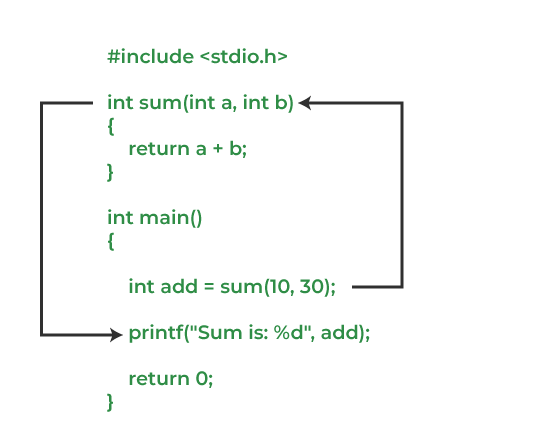Working of Function

## C

 `// C program to show function``// call and definition``#include ` `// Function that takes two parameters``// a and b as inputs and returns``// their sum``int` `sum(``int` `a, ``int` `b)``{``  ``return` `a + b;``}` `// Driver code``int` `main()``{``  ``// Calling sum function and``  ``// storing its value in add variable``  ``int` `add = sum(10, 30);``  ` `  ``printf``(``"Sum is: %d"``, add);``  ``return` `0;``}`

Output

`Sum is: 40`

## Types of Functions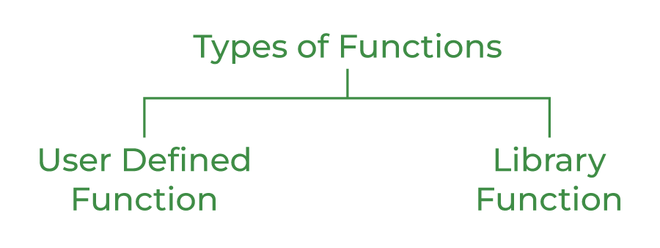Types of Functions in C

### 1. User Defined Function

Functions that are created by the programmer are known as User-Defined functions or “tailor-made functions”. User-defined functions can be improved and modified according to the need of the programmer. Whenever we write a function that is case-specific and is not defined in any header file, we need to declare and define our own functions according to the syntax.

• Changeable functions can be modified as per need.
• The Code of these functions is reusable in other programs.
• These functions are easy to understand, debug and maintain.

Example:

## C

 `// C program to show``// user-defined functions``#include ` `int` `sum(``int` `a, ``int` `b)``{``  ``return` `a + b;``}` `// Driver code``int` `main()``{``  ``int` `a = 30, b = 40;`` ` `  ``// function call``  ``int` `res = sum(a, b);` `  ``printf``(``"Sum is: %d"``, res);``  ``return` `0;``}`

Output

`Sum is: 70`

### 2. Library Function

A library function is also referred to as a “built-in function”. There is a compiler package that already exists that contains these functions, each of which has a specific meaning and is included in the package. Built-in functions have the advantage of being directly usable without being defined, whereas user-defined functions must be declared and defined before being used.

For Example:

`pow(), sqrt(), strcmp(), strcpy() etc.`

#### Advantages of C library functions

• C Library functions are easy to use and optimized for better performance.
• C library functions save a lot of time i.e, function development time.
• C library functions are convenient as they always work.

Example:

## C

 `// C program to implement``// the above approach``#include ``#include ` `// Driver code``int` `main()``{``  ``double` `Number;``  ``Number = 49;` `  ``// Computing the square root with``  ``// the help of predefined C``  ``// library function``  ``double` `squareRoot = ``sqrt``(Number);``  ` `  ``printf``(``"The Square root of %.2lf = %.2lf"``,``          ``Number, squareRoot);``  ``return` `0;``}`

Output

`The Square root of 49.00 = 7.00`

## Passing Parameters to Functions

The value of the function which is passed when the function is being invoked is known as the Actual parameters. In the below program 10 and 30 are known as actual parameters.

Formal Parameters are the variable and the data type as mentioned in the function declaration. In the below program, a and b are known as formal parameters.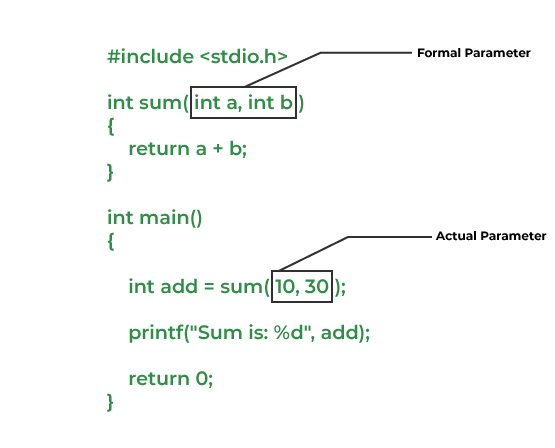1. Pass by Value: Parameter passing in this method copies values from actual parameters into function formal parameters. As a result, any changes made inside the functions do not reflect in the caller’s parameters.

Below is the C program to show pass-by-value:

## C

 `// C program to show use``// of call by value``#include ` `void` `swap(``int` `var1, ``int` `var2)``{``  ``int` `temp = var1;``  ``var1 = var2;``  ``var2 = temp;``}` `// Driver code``int` `main()``{``  ``int` `var1 = 3, var2 = 2;``  ``printf``(``"Before swap Value of var1 and var2 is: %d, %d\n"``,``          ``var1, var2);``  ``swap(var1, var2);``  ``printf``(``"After swap Value of var1 and var2 is: %d, %d"``,``          ``var1, var2);``  ``return` `0;``}`

Output

```Before swap Value of var1 and var2 is: 3, 2
After swap Value of var1 and var2 is: 3, 2```

2. Pass by Reference: The caller’s actual parameters and the function’s actual parameters refer to the same locations, so any changes made inside the function are reflected in the caller’s actual parameters.

Below is the C program to show pass-by-reference:

## C

 `// C program to show use of``// call by Reference``#include ` `void` `swap(``int` `*var1, ``int` `*var2)``{``  ``int` `temp = *var1;``  ``*var1 = *var2;``  ``*var2 = temp;``}` `// Driver code``int` `main()``{``  ``int` `var1 = 3, var2 = 2;``  ``printf``(``"Before swap Value of var1 and var2 is: %d, %d\n"``,``          ``var1, var2);``  ``swap(&var1, &var2);``  ``printf``(``"After swap Value of var1 and var2 is: %d, %d"``,``          ``var1, var2);``  ``return` `0;``}`

Output

```Before swap Value of var1 and var2 is: 3, 2
After swap Value of var1 and var2 is: 2, 3```

### Function Arguments and Return values

In C programming language, functions can be called either with or without arguments and might return values. They may or might not return values to the calling functions. To know more about function Arguments and Return values refer to the article – Function Arguments & Return Values in C.

• Function with no arguments and no return value
• Function with no arguments and with return value
• Function with argument and with no return value
• Function with arguments and with return value

My Personal Notes arrow_drop_up